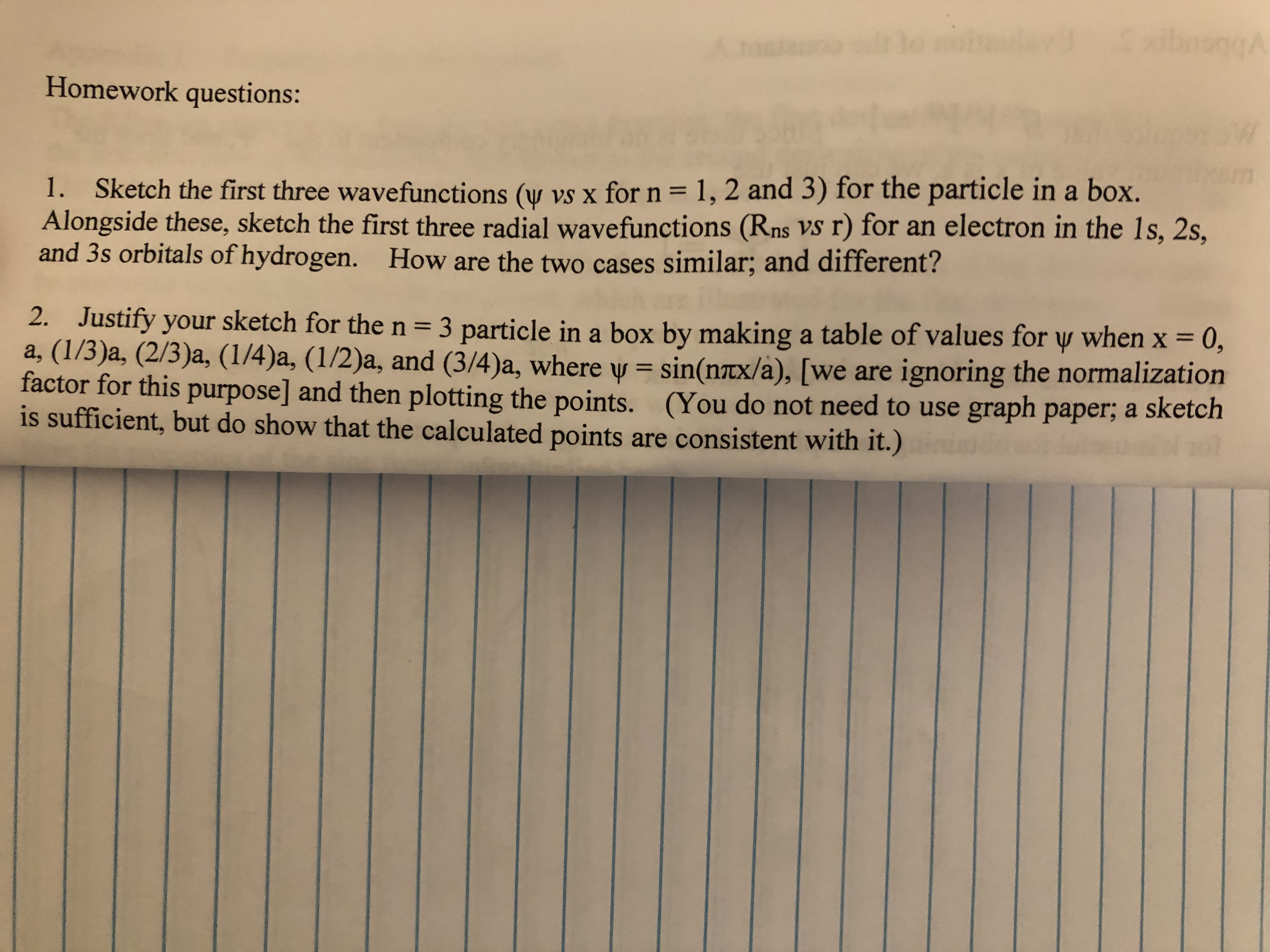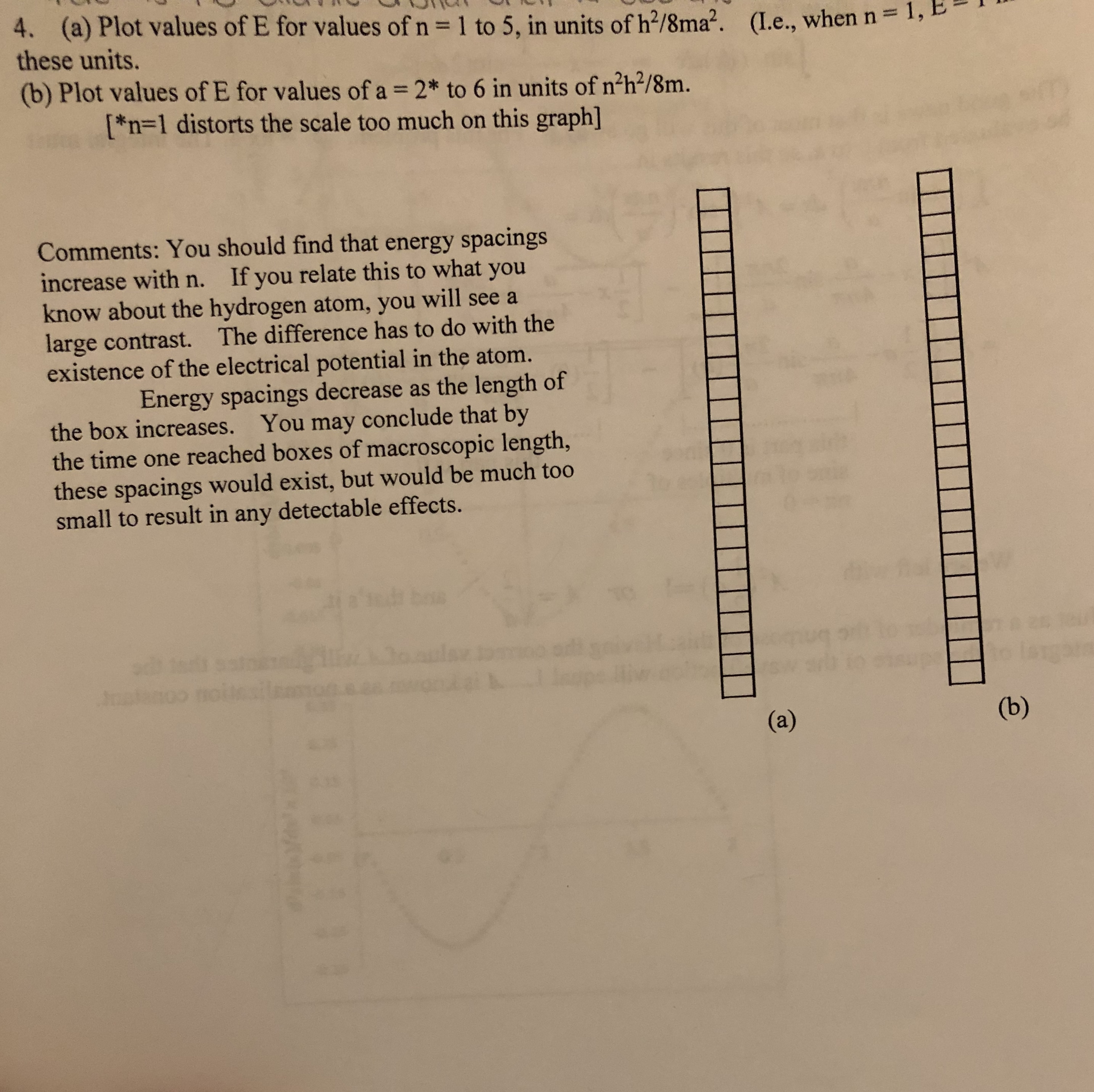# ibnongAHomework questions:1. Sketch the first three wavefunctions (w vs x for n = 1, 2 and 3) for the particle in a box.Alongside these, sketch the first three radial wavefunctions (Rns VS r) for an electron in the 1s, 2s,and 3s orbitals of hydrogen. How are the two cases similar; and different?%3D2. Justify your sketch for the n= 3 particle in a box by making a table of values for y when x = 0,a, (1/3)a, (2/3)a, (1/4)a, (1/2)a, and (3/4)a, where y = sin(ntx/a), [we are ignoring the normalizationfactor for this purpose] and then plotting the points. (You do not need to use graph paper; a sketchis sufficient, but do show that the calculated points are consistent with it.)%3D%3D%3D 4. (a) Plot values of E for values of n = 1 to 5, in units of h?/8ma?.these units.(L.e., when n= 1,(b) Plot values of E for values of a = 2* to 6 in units of n?h?/8m.[*n=1 distorts the scale too much on this graph]Comments: You should find that energy spacingsincrease with n. If you relate this to what youknow about the hydrogen atom, you will see alarge contrast. The difference has to do with theexistence of the electrical potential in the atom.Energy spacings decrease as the length ofthe box increases. You may conclude that bythe time one reached boxes of macroscopic length,these spacings would exist, but would be much toosmall to result in any detectable effects.(b)(a)1111

Question
34 views

I need help solving the problem. For 2) what value do I  use for a? 4) am I to use the tables labeled a) and b)? If so how?help_outlineImage TranscriptioncloseibnongA Homework questions: 1. Sketch the first three wavefunctions (w vs x for n = 1, 2 and 3) for the particle in a box. Alongside these, sketch the first three radial wavefunctions (Rns VS r) for an electron in the 1s, 2s, and 3s orbitals of hydrogen. How are the two cases similar; and different? %3D 2. Justify your sketch for the n= 3 particle in a box by making a table of values for y when x = 0, a, (1/3)a, (2/3)a, (1/4)a, (1/2)a, and (3/4)a, where y = sin(ntx/a), [we are ignoring the normalization factor for this purpose] and then plotting the points. (You do not need to use graph paper; a sketch is sufficient, but do show that the calculated points are consistent with it.) %3D %3D %3D fullscreenhelp_outlineImage Transcriptionclose4. (a) Plot values of E for values of n = 1 to 5, in units of h?/8ma?. these units. (L.e., when n= 1, (b) Plot values of E for values of a = 2* to 6 in units of n?h?/8m. [*n=1 distorts the scale too much on this graph] Comments: You should find that energy spacings increase with n. If you relate this to what you know about the hydrogen atom, you will see a large contrast. The difference has to do with the existence of the electrical potential in the atom. Energy spacings decrease as the length of the box increases. You may conclude that by the time one reached boxes of macroscopic length, these spacings would exist, but would be much too small to result in any detectable effects. (b) (a) 1111 fullscreen
check_circle

Step 1

Any value of ‘a’ can be taken to calculate ψ because the value of x is in terms of ‘a’, so the term ‘a’ will be canceled out and does not contribute in the calculation of ψ. The calculation of ψ for x = a is shown as fol...

### Want to see the full answer?

See Solution

#### Want to see this answer and more?

Solutions are written by subject experts who are available 24/7. Questions are typically answered within 1 hour.*

See Solution
*Response times may vary by subject and question.
Tagged in

### Quantum mechanics